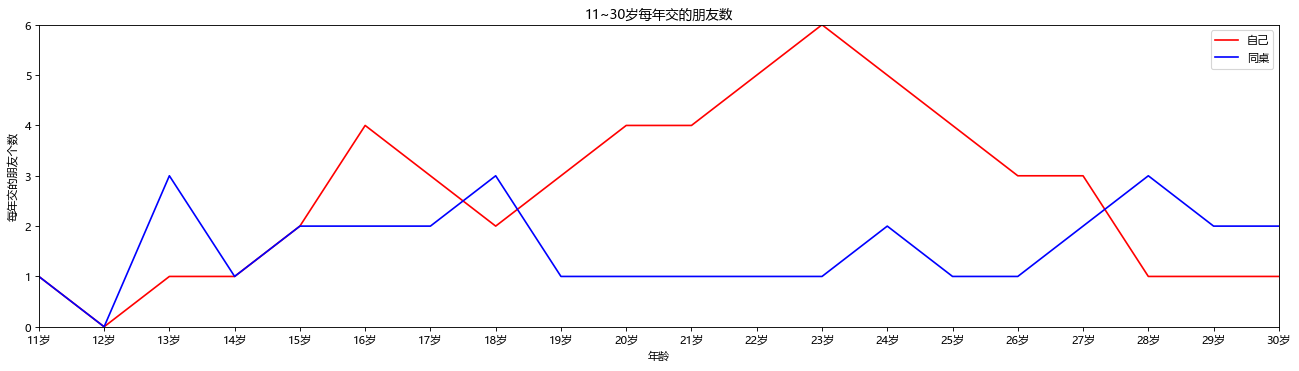### 本章节知识点

• 导入模块: import matplotlib.pyplot as plt/import numpy as np
• 定义图像窗口：plt.figure()
• 画图：plt.plot(x,y)
1. 定义坐标轴范围：plt.xlim()/plt.ylim()
2. 定义坐标轴名称：plt.xlabel()/plt.ylabel()
3. 定义坐标轴刻度及名称：plt.xticks()/plt.yticks()
4. 设置图像边框颜色：ax = plt.gca() / ax.spines[].set_color()
5. 调整刻度位置：ax.xaxis.set_ticks_position()/ax.yaxis.set_ticks_position()
6. 调整边框（坐标轴）位置：ax.spines[].set_position()
7. 添加图例：plt.legend()
8. 画点：plt.scatter()
9. 添加标注：plt.annotate()
10. 添加注释：plt.text()
11. 添加标题：plt.title()
• 设置格式：
1. 设置中文字体：plt.rcParams['font.family']='Microsoft YaHei'
2. 设置正常显示字符：plt.rcParams['axes.unicode_minus'] = False
一个图像层多个绘图区：plt.subplots()
import matplotlib.pyplot as plt
import numpy as np
import random


### 1. 创建两组数据

• 使用 np.linspace 定义x, 范围是 (-3,3) , 个数是50个数据, 产生一组均匀分布的50个数据, (x,y1)表示曲线1，(x,y2)表示曲线2。
x = np.linspace(-3, 3, 50)

y1 = 2 * x + 1
y2 = x ** 2


### 2. 定义图像窗口并画图

1. 在画图前使用plt.figure()定义一个图像窗口:
编号为3; 大小为(8, 5)；这两项参数可缺省。其中, num参数决定了程序运行后弹出的图像窗口名字。
2. 接着,我们使用plt.plot画出(x ,y2)曲线；使用plt.plot画(x ,y1)曲线;
3. 曲线的颜色属性(color)为红色
4. 曲线的宽度(linewidth)为1.0
5. 曲线的类型(linestyle)为虚线, 除了虚线外, 还可使用以下线性：'-'、'--'、'-.'、':' 。
接着，我们使用plt.show()显示图像。
# 定义一个图像窗口
plt.figure()
# 分别绘制 y1, y2图像
plt.plot(x, y1)
plt.plot(x, y2, color='red', linewidth=1.0, linestyle='--')
plt.show()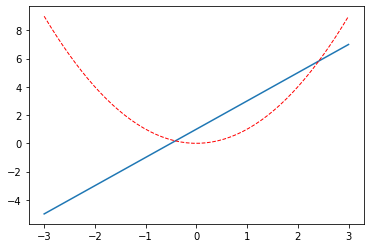# 创建画布
fig, ax = plt.subplots()
ax.plot(x, y1, color='red', linewidth=1.0, linestyle='--')
ax.plot(x, y2)
plt.show()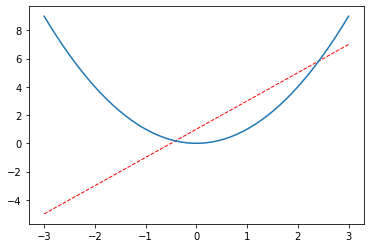### 3. 添加图例

• 任务 : 为两条线添加图例, 图例位置在右上角
• 设置两条线的类型等信息（蓝色实线与红色虚线), 并且通过label参数为两条线设置名称。
1. 比如直线的名称就叫做 "linear line", 曲线的名称叫做 "square line"。
2. 只是设置好名称并不能使我们的图例出现，要通过plt.legend()设置图例的显示。
3. legend获取代码中的 label 的信息, plt就能自动的为我们添加图例。
• 或者设置 handles 参数来选择图例中显示的内容。
1. 在代码 plt.plot(x, y2, label='linear line') 和 plt.plot(x, y1, label='square line') 中用变量l1和l2分别存储起来,
2. 而且需要注意的是 l1, l2, 要以逗号结尾, 因为plt.plot() 返回的是一个列表。然后将 l1,l2 这样的objects以列表的形式传递给 handles。
3. label 参数可以用来单独修改之前的 label 信息, 给不同类型的线条设置图例信息。
1. 如果希望图例能够更加个性化，可通过以下方式更改：参数 loc 决定了图例的位置，比如参数 loc='upper right' 表示图例将添加在图中的右上角。
其中’loc’参数有多种，’best’表示自动分配最佳位置，其余的如下：
'best' : 0,
'upper right' : 1,
'upper left' : 2,
'lower left' : 3,
'lower right' : 4,
'right' : 5,
'center left' : 6,
'center right' : 7,
'lower center' : 8,
'upper center' : 9,
'center' : 10

#### 3.1 添加图例 : 方式一

# 方式一 :
plt.plot(x, y1, label='linear line')
plt.plot(x, y2, color='red', linewidth=1.0, linestyle='--', label='square line')
# 设置图例的位置在右上角
plt.legend(loc='upper right')

<matplotlib.legend.Legend at 0x25c685f1b48>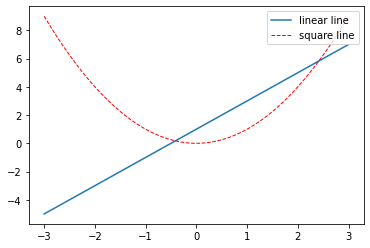#### 3.2 添加图例 : 方式二

# l1, l2, 要以逗号结尾, 因为plt.plot() 返回的是一个列表
l1, = plt.plot(x, y1)
l2, = plt.plot(x, y2, color='red', linewidth=1.0, linestyle='--')

# 设置图例
plt.legend(handles=[l1, l2, ], labels=['linear line', 'square line'], loc='upper right')

<matplotlib.legend.Legend at 0x25c685b3c08>### 4. 定义坐标轴范围

• 定义x轴名称为X，范围为（-1,2），y轴名称为Y，范围为（-2,3）
1. 使用plt.xlim设置x坐标轴范围：(-1, 2)；
2. 使用plt.ylim设置y坐标轴范围：(-2, 3)；
3. 使用plt.xlabel设置x坐标轴名称：'x'
4. 使用plt.ylabel设置y坐标轴名称：'y'
# 定义一个图像窗口
# num 是图像的唯一标识, figsize : 设置宽高
plt.figure(num=3, figsize=(8, 5))

plt.plot(x, y1, label='linear line')
plt.plot(x, y2, color='red', linewidth=1.0, linestyle='--', label='square line')

plt.xlim((-1, 2))
plt.xlim((-2, 3))

plt.xlabel('X')
plt.ylabel('Y')
plt.show()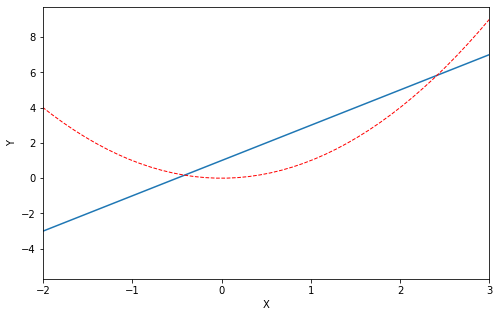### 5. 设置坐标轴刻度

• 设置x轴刻度：

1. 范围是(-1,2)
2. 个数是5
• 有时候，我们的坐标轴刻度可能并不是一连串的数字, 而是一些文字，或者我们想要调整坐标轴的刻度的稀疏，这时，就需要使用plt.xticks()或者plt.yticks()来进行调整：

1. 首先, 使用np.linspace定义新刻度范围以及个数: 范围是(-1,2); 个数是5。
2. 使用plt.xticks设置x轴刻度：范围是(-1,2) , 个数是5。
3. 使用plt.yticks设置y轴刻度以及名称：刻度为[-2, -1.8, -1, 1.22, 3]
5. 使用plt.show()显示图像。
# 定义图像窗口
plt.figure(num=3, figsize=(8, 5))
# 绘制 x-y1 和 x-y2 的图像
plt.plot(x, y1, label='linear line')
plt.plot(x, y2, color='red', linewidth=1.0, linestyle='--', label='square line')

# 设置图例显示, 必须设置图的 label , 图例才能显示出来
plt.legend(loc='upper right')

# 设置 x轴和y轴的范围
plt.xlim((-1, 2))
plt.ylim((-2, 3))

# 设置 x 轴和 y 轴的标签
plt.xlabel('X')
plt.ylabel('Y')

# 设置刻度
new_ticks = np.linspace(-1, 2, 5)
print(new_ticks)

plt.xticks(new_ticks)
# 将数值刻度替换为字符串刻度
plt.yticks([-2, -1.8, -1, 1.22, 3], [r'$really\ bad$', r'$bad$', r'$normal$', r'$good$', r'$really\ good$'])

plt.show()

[-1.   -0.25  0.5   1.25  2.  ]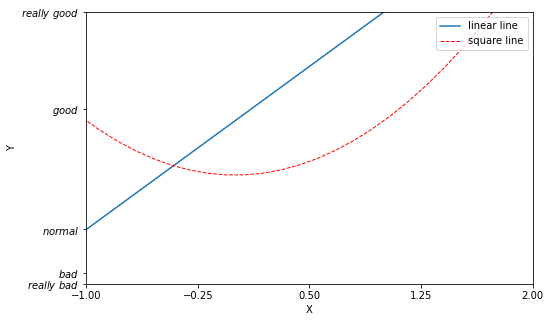### 6. 设置图像边框

• 获取坐标轴信息，设置图像边框颜色，取消上边框和右边框
• 上图中坐标轴总是由上下左右四条线组成，我们也可以对它们进行修改：
1. 使用 ax = plt.gca() 获取当前坐标轴信息。
2. 使用 ax.spines['right']设置边框：右侧边框；
3. 使用 .set_color设置边框颜色：默认白色；
4. 使用 .spines设置边框：上边框；
5. 使用 .set_color设置边框颜色：默认白色；
# 绘制图像
plt.figure(num=3, figsize=(8, 5), )
plt.plot(x, y1, label='linear line')
plt.plot(x, y2, color='red', linewidth=1.0, linestyle='--', label='square line')

# 获取坐标轴信息
ax = plt.gca()

# 取消右边框(设置右边框颜色为 none)
ax.spines['right'].set_color('none')
# 取消上边框
ax.spines['top'].set_color('none')
plt.show()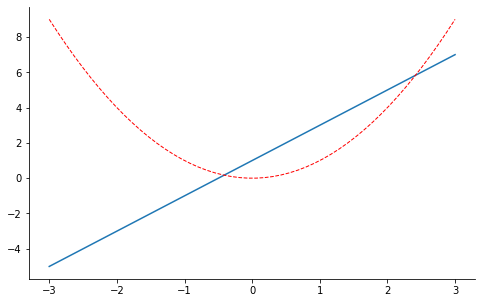### 7. 移动x轴的的位置

• 将 xy 轴的位置移动到 0 点
1. 使用.xaxis.set_ticks_position设置x坐标刻度数字或名称的位置：bottom.（所有位置：top，bottom, both, default, none）;
2. 使用.spines设置边框：x轴;
3. 使用.set_position设置边框位置：y=0的位置；（位置所有属性：outward，axes，data）
4. 使用.yaxis.set_ticks_position设置y坐标刻度数字或名称的位置：left.（所有位置：left，right，both，default，none）
5. 使用.spines设置边框：y轴；
6. 使用.set_position设置边框位置：x=0的位置；（位置所有属性：outward，axes，data）
7. 使用plt.show显示图像.
# 绘制图像
plt.figure(num=3, figsize=(8, 5))
plt.plot(x, y2)
plt.plot(x, y1, color='red', linewidth=1.0, linestyle='--')

# 获取坐标轴信息
ax = plt.gca()
# 设置右边框为白色(不显示)
ax.spines['right'].set_color('none')
ax.spines['top'].set_color('none')

# 移动x坐标轴刻度或者名称的位置
ax.xaxis.set_ticks_position('bottom')
# 设置边框位置: y=0的位置
ax.spines['bottom'].set_position(('data', 0))
# 移动y坐标轴或者名称的位置
ax.yaxis.set_ticks_position('left')
# 设置边框位置: x=0的位置
ax.spines['left'].set_position(('data', 0))
plt.show()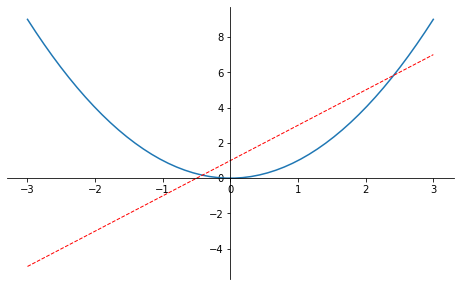### 8. 在坐标轴上画图并标识点的位置

• 在坐标轴上画出y=2x+1的图，并用点标注出（1,3）的位置
• 当图线中某些特殊地方需要标注时，我们可以使用 annotation. matplotlib 中的 annotation 有两种方法
1. 一种是用 plt 里面的 annotate
2. 一种是直接用 plt 里面的 text 来写标注
• 标注出点(x0, y0)的位置信息
1. plt.plot([x0, x0,], [0, y0,], 'k--', linewidth=2.5) 画出一条垂直于x轴的虚线。其中，[x0, x0,], [0, y0,] 表示在图中画一条从点 (x0,y0) 到 (x0,0) 的直线，'k--' 表示直线的颜色为黑色(black)，线形为虚线
2. plt.scatter 函数可以在图中画点，此时我们画的点为 (x0,y0), 点的大小(size)为 50， 点的颜色为蓝色(blue),可简写为 b。
# 准备数据
x = np.linspace(-3, 3 , 50)
y = 2 * x + 1

# 绘制图像
plt.figure(num=1, figsize=(8,5))
plt.plot(x, y, color='blue', linewidth=1.0)

ax = plt.gca()
# 取消上边框和右边框
ax.spines['right'].set_color('none')
ax.spines['top'].set_color('none')

ax.xaxis.set_ticks_position('bottom')
# 移动x轴到y=0的位置
ax.spines['bottom'].set_position(('data', 0))
ax.yaxis.set_ticks_position('left')
# 移动y轴到x=0的位置
ax.spines['left'].set_position(('data', 0))

# 标点
x0 = 1
y0 = 2 * x0 + 1
plt.scatter([x0, ] , [y0, ], s=50, color='r')
plt.show()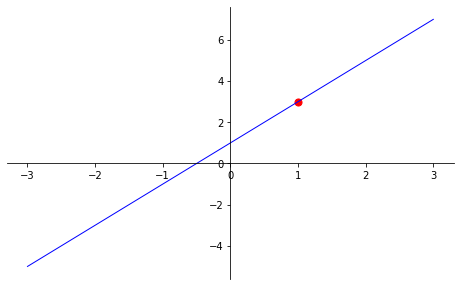### 9. 绘制上海指定时段温度变化

• 准备数据并画图——上海在11点到12点一小时内每分钟的温度（15度-18度）变化
# 准备数据
x = range(60)
# 随机生成 60 个范围在 15 ~ 18 的随机数
y_shanghai = [random.uniform(15,18) for i in x]
# 创建画布 (dpi = 图像每英寸长度内的像素点数)
plt.figure(figsize=(20,8), dpi=80)
plt.plot(x, y_shanghai)
plt.show()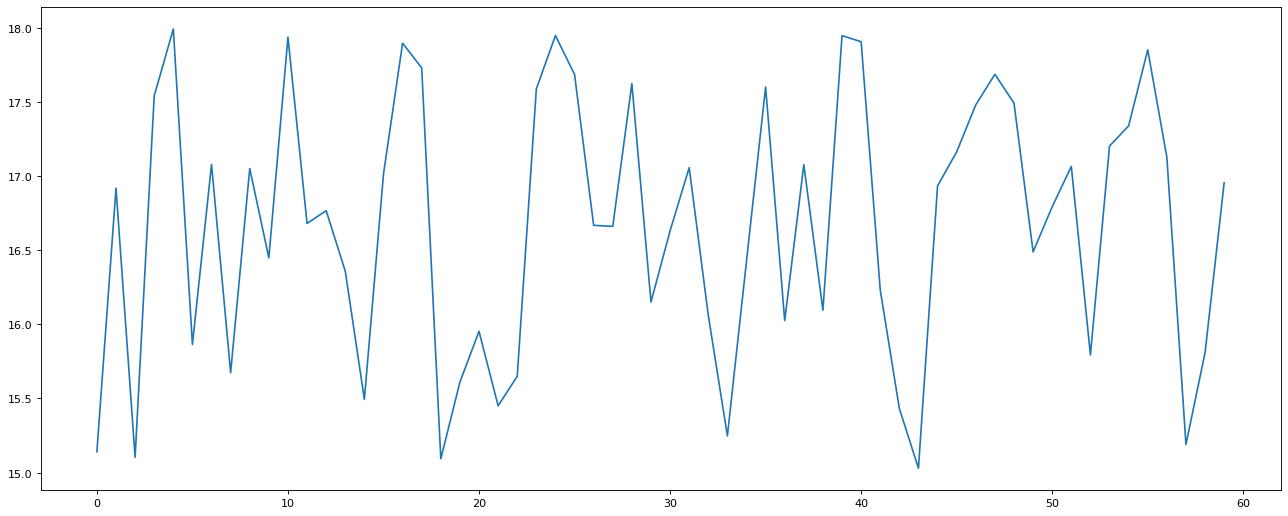### 10. 修改x刻度样式

• x刻度改为11点()分格式
• 切片操作基本表达式：object[start_index:end_index:step]
plt.figure(figsize=(20,8),dpi=80)
# 设置中文字体
plt.rcParams['font.family'] = 'Microsoft YaHei'

# 生成数据
x = range(60)
y = [random.uniform(15,18) for i in x]
# 生成x轴刻度
x_label = ["11点{}分".format(i) for i in x]
# 设置x轴刻度
# 切片操作基本表达式：object[start_index:end_index:step]
plt.xticks(x[::5], x_label[::5])
plt.plot(x, y_shanghai)
plt.show()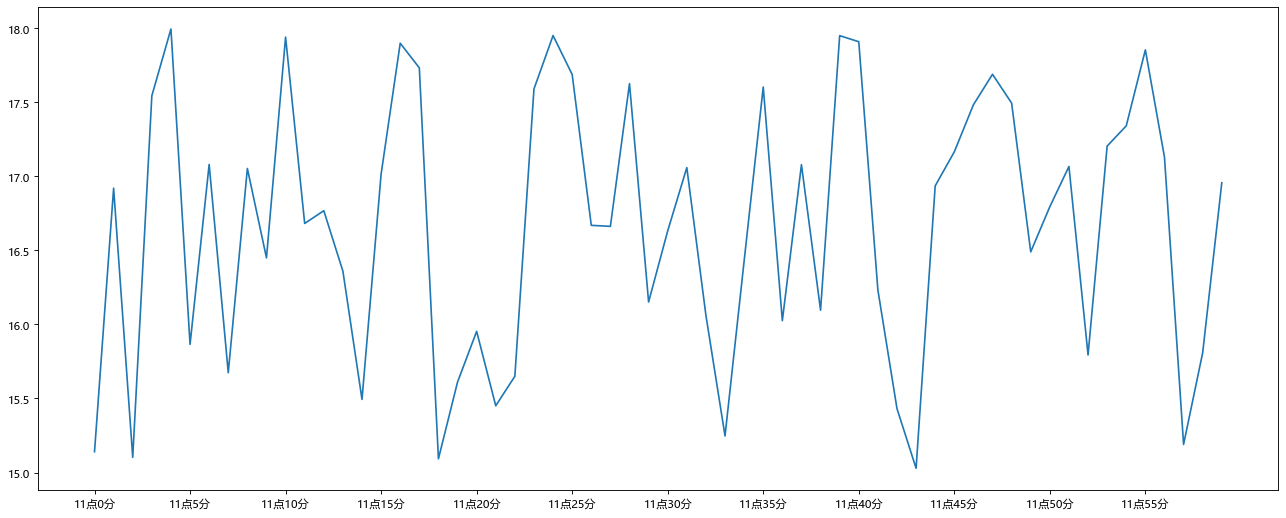### 11. 添加文字标题和xy轴的文字描述

x = range(60)
y_shanghai = [random.uniform(15,18) for i in x ]
plt.figure(figsize=(20,8), dpi=80)
x_label = ["11点{}分".format(i) for i in x]
plt.xticks(x[::5], x_label[::5])

plt.xlabel("时间变化")
plt.ylabel("温度变化")
plt.title("某城市一小时内每分钟的温度变化")
plt.plot(x, y_shanghai)
plt.show()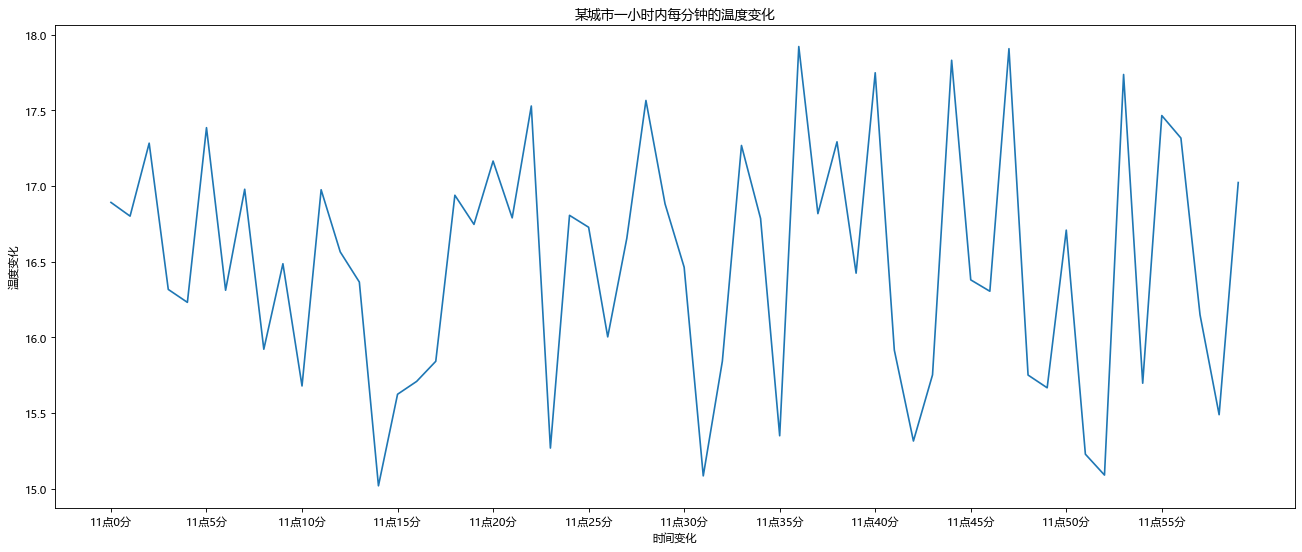### 12. 添加新的折线

• 再添加一个城市的温度变化，例北京当时的温度为10度到14度
# 创建数据
x = range(60)
y_shanghai = [random.uniform(15,18) for i in x]
y_beijing = [random.uniform(10,14) for i in x]

# 创建画布
plt.figure(figsize=(20,8), dpi=80)
# 绘制图层
plt.plot(x, y_shanghai, color='r', linestyle='--')
plt.plot(x, y_beijing, color='b')

plt.show()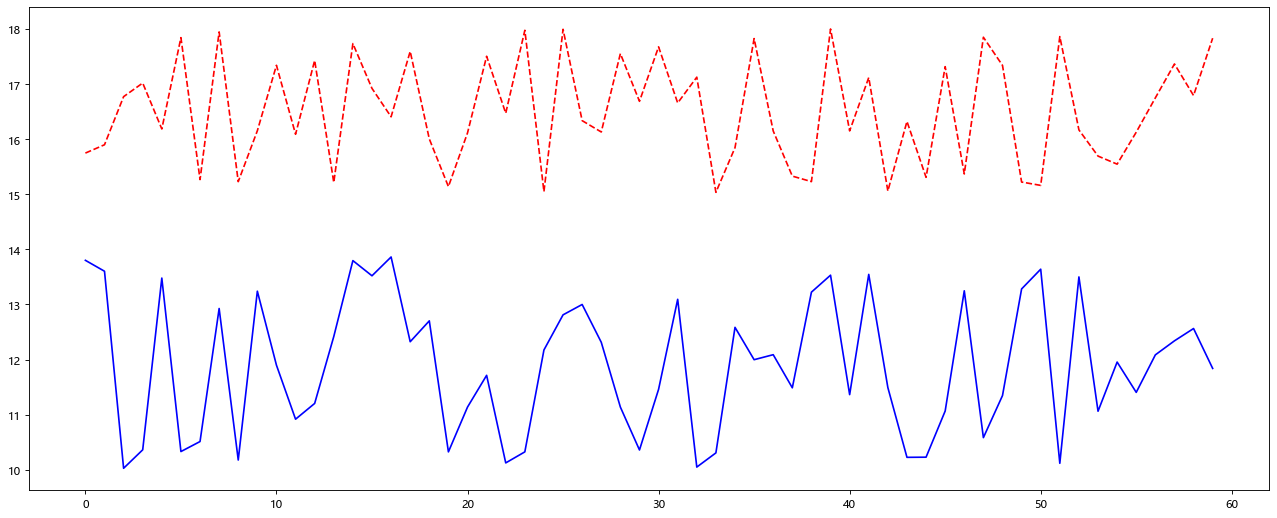### 12. 显示图例并修改刻度

• 显示图例
• 修改x轴刻度为11点()分
• 添加标题和x轴的描述
# 创建数据
x = range(60)
x_label = ["11点{}分".format(i) for i in x]
y_shanghai = [random.uniform(15,18) for i in x]
y_beijing = [random.uniform(10,14) for i in x]

# 设置显示中文
plt.rcParams['font.family'] = 'Microsoft YaHei'
# 创建画布
plt.figure(figsize=(20,8), dpi=80)
# 绘制图层
plt.plot(x, y_shanghai, color='r', linestyle='--', label='上海')
plt.plot(x, y_beijing, color='b', label='北京')
# 设置刻度
plt.xticks(x[::5], x_label[::5])
# 设置图例
plt.legend(loc='best')
#添加描述信息
plt.xlabel("时间变化")
plt.ylabel("温度变化")
plt.title("上海，北京两地11点到12点每分钟的温度变化状况")
plt.show()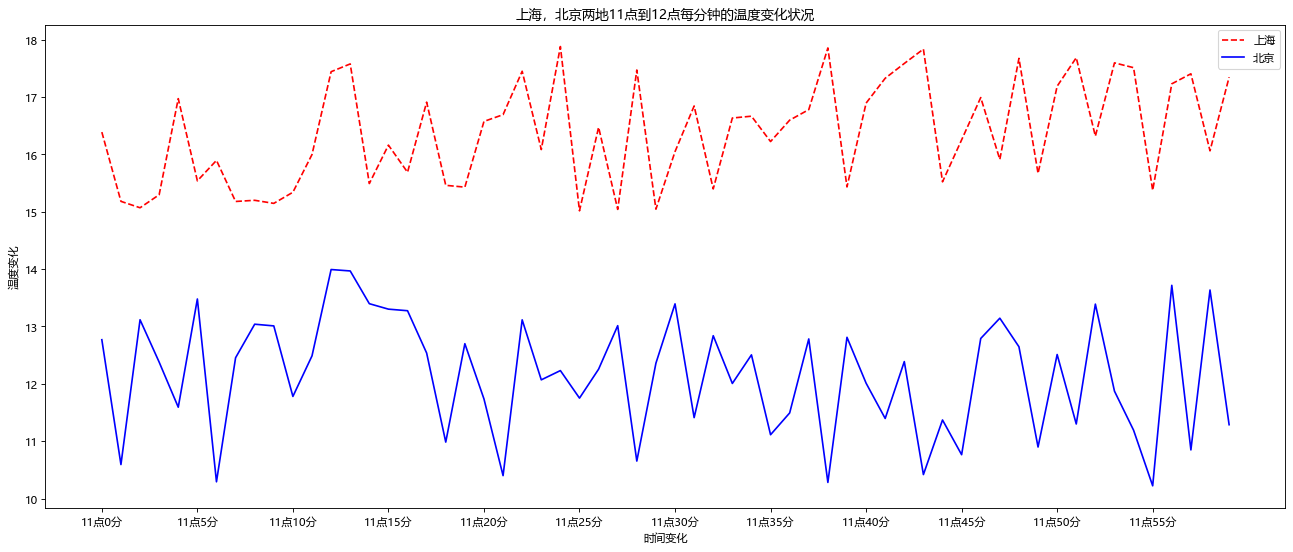### 13. 作业

#### 1. 作业一 :

• 画出直线 y = x-1, 线型为虚线，线宽为1，纵坐标范围（-2，1），横坐标范围（-1，2），横纵坐标在（0，0）坐标点相交。横坐标的 [-1,-0.5,1] 分别对应 [bad, normal, good]
# 生成数据
x = np.linspace(-3,3,50)
y = x - 1

# 绘制图形
plt.figure(figsize=(8,5), num=2)
# 设置横坐标范围
plt.xlim((-1,2))
# 设置纵坐标范围
plt.ylim((-2,1))

# 修改xy坐标轴位置到 0
ax = plt.gca()
# 清除右侧和上方的边框
ax.spines['right'].set_color('none')
ax.spines['top'].set_color('none')
# 移动x轴和y轴的位置到 0 点
ax.xaxis.set_ticks_position('bottom')
ax.spines['bottom'].set_position(('data',0))
ax.yaxis.set_ticks_position('left')
ax.spines['left'].set_position(('data', 0))

# 绘制图像
plt.plot(x, y, color='r', linestyle='--')
# 设置刻度
# plt.xticks([-1,-0.5,1], [r'$bad$', r'$normal$', r'$good$'])

plt.show()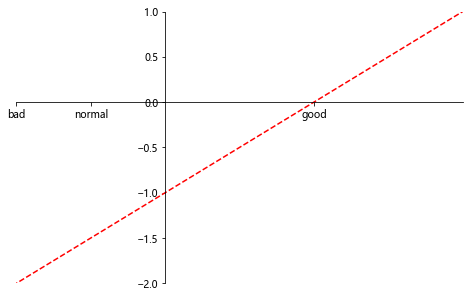#### 2. 作业二

• 假设大家在30岁的时候，根据自己的实际情况，统计出来了你和同桌从11岁到30岁每年交的朋友的数量如列表a和b,请绘制出该数据的折线图,以便分析自己和同桌每年交朋友的数量走势
1. a = [1,0,1,1,2,4,3,2,3,4,4,5,6,5,4,3,3,1,1,1]
2. b = [1,0,3,1,2,2,2,3,1,1,1,1,1,2,1,1,2,3,2,2]
3. y轴表示个数
4. x轴表示岁数,比如11岁,12岁
# 创建数据
y1 = [1,0,1,1,2,4,3,2,3,4,4,5,6,5,4,3,3,1,1,1]
y2 = [1,0,3,1,2,2,2,3,1,1,1,1,1,2,1,1,2,3,2,2]

x = range(11,31)
x_label = ["{}岁".format(i) for i in x]

# 定义图像窗口
plt.figure(figsize=(20,5), dpi=80)
# 设置中文字体
plt.rcParams['font.family'] = 'Microsoft YaHei'
# 设置y轴范围
plt.ylim((0,6))
plt.xlim((11,29))
# 设置x轴刻度
plt.xticks(x, x_label)
# 设置标题以及详细信息
plt.xlabel('年龄')
plt.ylabel('每年交的朋友个数')
plt.title('11~30岁每年交的朋友数')

# 绘制图像
plt.plot(x, y1, color='r', label='自己')
plt.plot(x, y2, color='b', label='同桌')
# 显示图例
plt.legend(loc='best')

plt.show()